# Properties of Graph Data Structure

### <<Previous Next >>

In this page, we will learn about various properties of graph

### Graph Properties

Adjacent Vertices(or) Neighbors: If there is an edge between two vertices, then these two vertices are said to be adjacent vetices. For example, from the graph below, A and B are adjacent vertices, but A and D are not.

Path: Path is the sequence of vertices in which each vertex is adjacent to next one. In the above graph, A, B, C, E is one path and A, B, D, E is another path.

Cycle: is a path consisting of, at least 3 vertices that starts and ends with the same vertex.

Loop: is a special case of cycle, in which a single arc begins and ends with same vertex.

Connected: If there is a path between two vertices, then we say that two vertices are connected

# Properties of Graph Data Structure

### <<Previous Next >>

In this page, we will learn about various properties of graph

### Graph Properties

Adjacent Vertices(or) Neighbors: If there is an edge between two vertices, then these two vertices are said to be adjacent vetices. For example, from the graph below, A and B are adjacent vertices, but A and D are not.

Path: Path is the sequence of vertices in which each vertex is adjacent to next one. In the above graph, A, B, C, E is one path and A, B, D, E is another path.

Cycle: is a path consisting of, at least 3 vertices that starts and ends with the same vertex.

Loop: is a special case of cycle, in which a single arc begins and ends with same vertex.

Connected: If there is a path between two vertices, then we say that two vertices are connected

### << Previous Next >>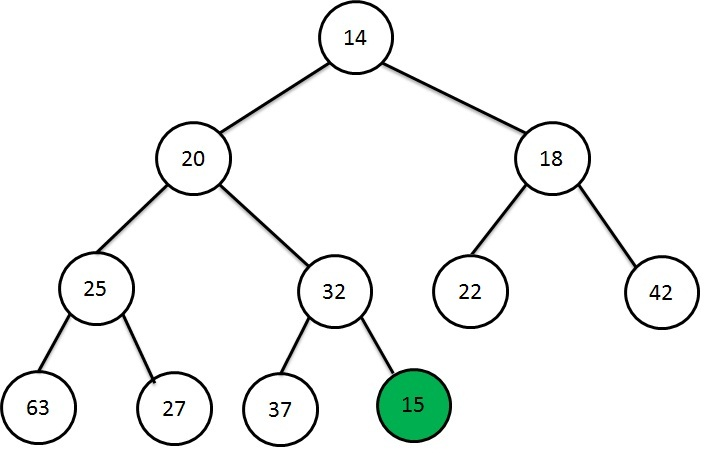Heap Data Structure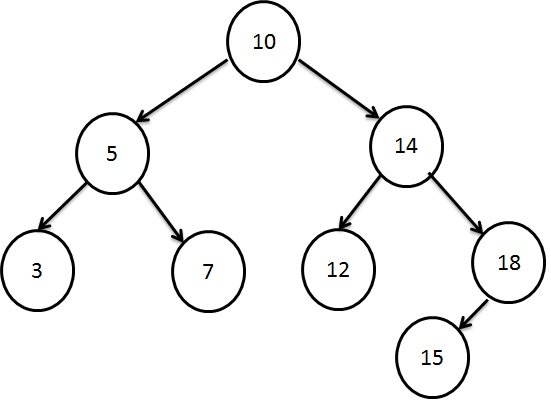Searching using Binary Search Tree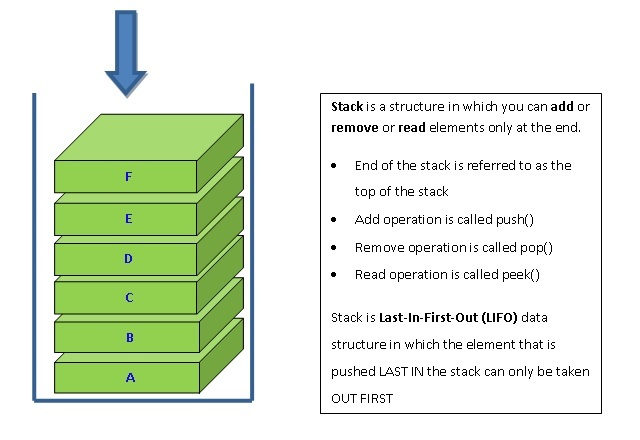Stacking the Data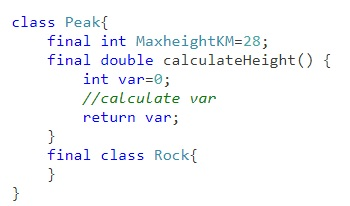Learn Java final keyword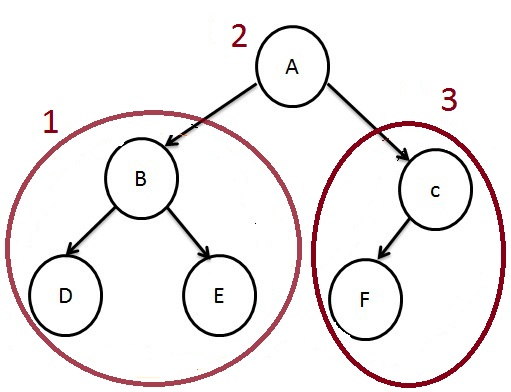How to traverse a Tree?